# Heatmap and Annotation Legends

Author: Zuguang Gu ( z.gu@dkfz.de )

Date: 2017-04-24

The legends for heatmaps are composed with a color bar, labels and titles. ComplexHeatmap automatically generates legends according to the input matrix and annotations, while also provide flexibility to customize and add new legends.

## Basic settings

Legends for all heatmaps and row annotations are drawn together and legends for all column annotations are drawn together. The legends for heatmaps and legends for annotations are put in independent viewports.

``````library(ComplexHeatmap)
library(circlize)

set.seed(123)
mat = matrix(rnorm(80, 2), 8, 10)
mat = rbind(mat, matrix(rnorm(40, -2), 4, 10))
rownames(mat) = paste0("R", 1:12)
colnames(mat) = paste0("C", 1:10)

ha_column = HeatmapAnnotation(df = data.frame(type1 = c(rep("a", 5), rep("b", 5))),
col = list(type1 = c("a" =  "red", "b" = "blue")))
ha_row = rowAnnotation(df = data.frame(type2 = c(rep("A", 6), rep("B", 6))),
col = list(type2 = c("A" =  "green", "B" = "orange")), width = unit(1, "cm"))

ht1 = Heatmap(mat, name = "ht1", column_title = "Heatmap 1", top_annotation = ha_column)
ht2 = Heatmap(mat, name = "ht2", column_title = "Heatmap 2")
ht_list = ht1 + ht2 + ha_row

draw(ht_list)
``````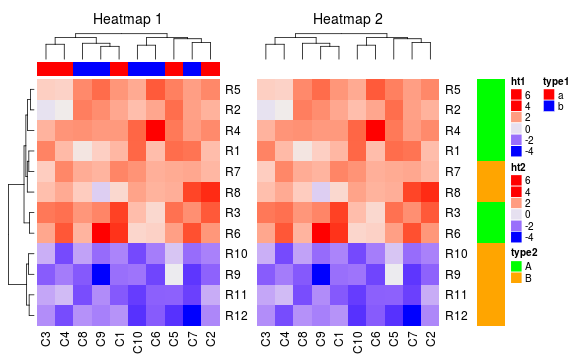Side of legends can be set by `heatmap_legend_side` and `annotation_legend_side`.

``````draw(ht_list, heatmap_legend_side = "left", annotation_legend_side = "bottom")
``````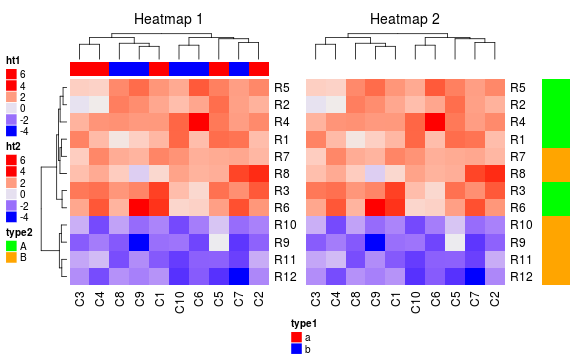`show_heatmap_legend` and `show_annotation_legend` set visibility of legends.

``````draw(ht_list, show_heatmap_legend = FALSE, show_annotation_legend = FALSE)
``````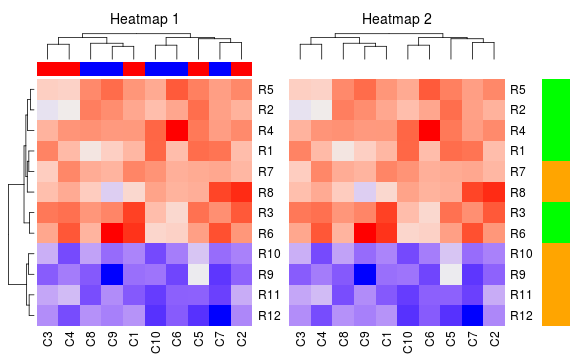You can choose to only show some of the heatmap legends by setting `show_heatmap_legend` to a logical value when constructing single heatmaps. Also `HeatmapAnnotation()` (or the shortcut function `columnAnnotation()` and `rowAnnotation()`) provides `show_legend` argument to control visibility of annotation legends.

``````ha_column = HeatmapAnnotation(df = data.frame(type1 = c(rep("a", 5), rep("b", 5))),
col = list(type1 = c("a" =  "red", "b" = "blue")), show_legend = FALSE)
ha_row = rowAnnotation(df = data.frame(type2 = c(rep("A", 6), rep("B", 6))),
col = list(type2 = c("A" =  "green", "B" = "orange")), show_legend = FALSE, width = unit(1, "cm"))

ht1 = Heatmap(mat, name = "ht1", column_title = "Heatmap 1", top_annotation = ha_column)
ht2 = Heatmap(mat, name = "ht2", column_title = "Heatmap 2", show_heatmap_legend = FALSE)
ht1 + ht2 + ha_row
``````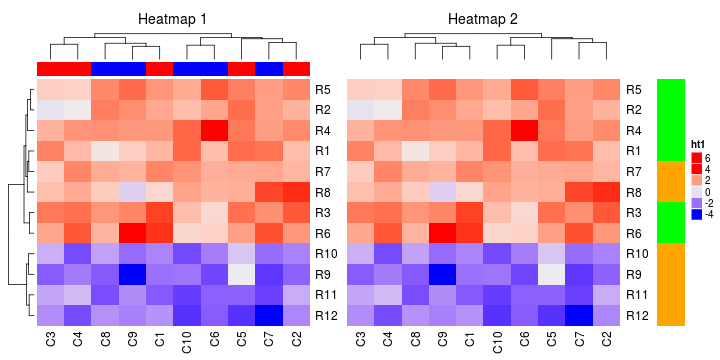## Customization of legends

Legend itself can be flexibly customized. Parameters for making the legend can be set by `heatmap_legend_param` (for heatmap) or `annotation_legend_param` (for annotations). The parameters that can be set are as follows:

• `title`: title of the legend
• `title_gp`: graphic parameters for the legend title
• `title_position`: position of title relative to the legend, possible values are `topcenter`, `topleft`, `leftcenter`, `lefttop`.
• `color_bar`: style of the color bar, i.e. continuous or discrete
• `grid_height`: height of the small grid in the color bar, only works for discrete color bar
• `grid_width`: width of the color bar
• `grid_border`: border of the color bar
• `at`: break values shown on the legend
• `labels`: labels which correspond to the breaks values
• `labels_gp`: graphic parameters for legend labels
• `nrow` and `ncol`: if there are too many legends, they can be put into an array. These two parameters controls number of rows or columns
• `legend_direction`: when `color_bar` is set to `continuous`, it controls the direction of the legend, possible values are `vertical` and `horizontal`
• `legend_width` and `legend_height`: when `color_bar` is `continuous`, the width or height of the legend

Following example changes the default graphic parameters for labels and titles:

``````df = data.frame(type = c(rep("a", 5), rep("b", 5)))
ha = HeatmapAnnotation(df = df, col = list(type = c("a" =  "red", "b" = "blue")),
annotation_legend_param = list(type = list(title = "TYPE", title_gp = gpar(fontsize = 14),
labels_gp = gpar(fontsize = 8))))
ht1 = Heatmap(mat, name = "ht1", column_title = "Heatmap 1", top_annotation = ha)
ht2 = Heatmap(mat, name = "ht2", column_title = "Heatmap 2",
heatmap_legend_param = list(title = "Heatmap2", title_gp = gpar(fontsize = 8),
labels_gp = gpar(fontsize = 14)))
ht1 + ht2
``````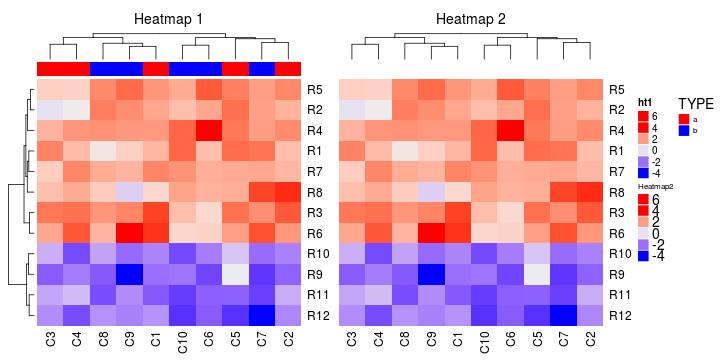You can specify break values and break labels (both for character values and numeric values) by `at` and `labels` in corresponding `heatmap_legend_param` and `annotation_legend_param`. Note `at` can also be character break values.

``````ha = HeatmapAnnotation(df = df, col = list(type = c("a" =  "red", "b" = "blue")),
annotation_legend_param = list(type = list(title = "TYPE", title_gp = gpar(fontsize = 14),
labels_gp = gpar(fontsize = 8), at = c("a", "b"), labels = c("A", "B"))))
ht1 = Heatmap(mat, name = "ht1", column_title = "Heatmap 1", top_annotation = ha,
heatmap_legend_param = list(at = c(-3, 0, 3), labels = c("-three", "zero", "+three")))
ht1 + ht2
``````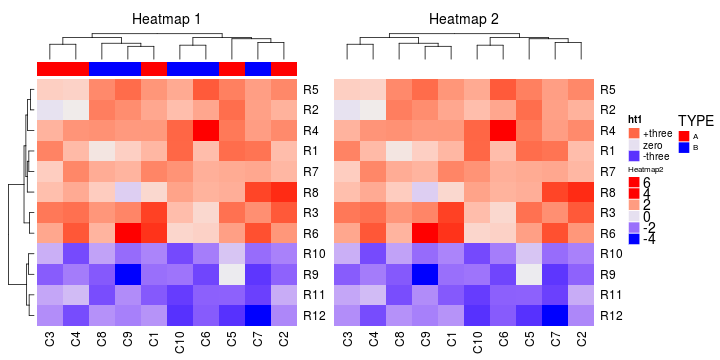If you have many levels in your annotation or matrix, you can put all levels into an array by specifying `nrow` or `ncol`:

``````ha_chr = rowAnnotation(chr = sample(paste0("chr", 1:20), nrow(mat), replace = TRUE),
annotation_legend_param = list(chr = list(ncol = 2, title = "chromosome", title_position = "topcenter")),
width = unit(5, "mm"))
ht1 = Heatmap(mat, name = "ht1")
ht1 + ha_chr
``````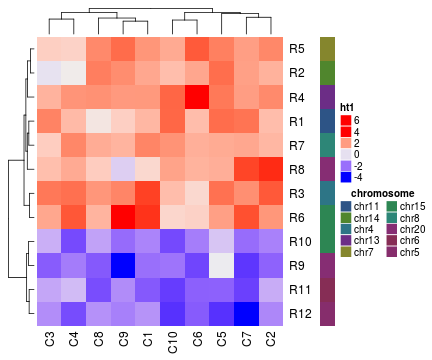Or put at bottom of the heatmap:

``````ha_chr = rowAnnotation(chr = sample(paste0("chr", 1:20), nrow(mat), replace = TRUE),
annotation_legend_param = list(chr = list(nrow = 2, title = "chr", title_position = "leftcenter")),
width = unit(5, "mm"))
ht1 = Heatmap(mat, name = "ht1", show_heatmap_legend = FALSE)
draw(ht1 + ha_chr, heatmap_legend_side = "bottom")
``````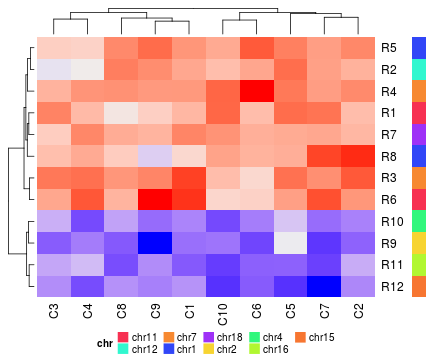If you don't like the default discrete color bar for continuous values, you can specify `color_bar` to `continuous`. For the simple annotation which contains continuous values, `color_bar` can also be set to `continuous`.

``````ha = HeatmapAnnotation(df = data.frame(value = runif(10)),
col = list(value = colorRamp2(c(0, 1), c("white", "blue"))),
annotation_legend_param = list(color_bar = "continuous", at = c(0, 0.5, 1),
labels = c("low", "median", "high"), legend_height = unit(4, "cm")))
Heatmap(mat, name = "ht1", top_annotation = ha, heatmap_legend_param = list(color_bar = "continuous"))
``````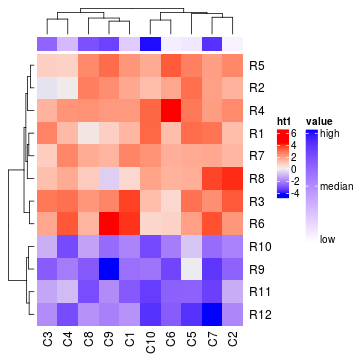Some users prefer to put the legend at the bottom of heatmaps.

``````ht = Heatmap(mat, name = "ht1", heatmap_legend_param = list(color_bar = "continuous", legend_direction = "horizontal",
legend_width = unit(5, "cm"), title_position = "lefttop"))
draw(ht, heatmap_legend_side = "bottom")
``````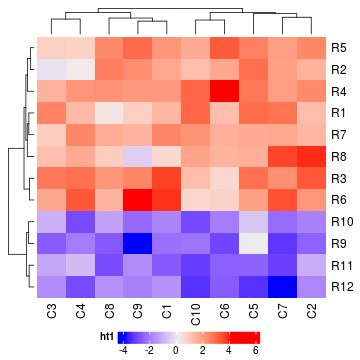If you want to change default settings for all heatmaps/annotations, you can set it globally by `ht_global_opt()`.

``````ht_global_opt(heatmap_legend_title_gp = gpar(fontsize = 16), annotation_legend_labels_gp = gpar(fontface = "italic"))
ha = HeatmapAnnotation(df = data.frame(value = runif(10)),
col = list(value = colorRamp2(c(0, 1), c("white", "blue"))),
annotation_legend_param = list(color_bar = "continuous"))
ht1 = Heatmap(mat, name = "ht1", column_title = "Heatmap 1", top_annotation = ha)
ht2 = Heatmap(mat, name = "ht2", column_title = "Heatmap 2", heatmap_legend_param = list(title_gp = gpar(fontsize = 8)))
ht1 + ht2
``````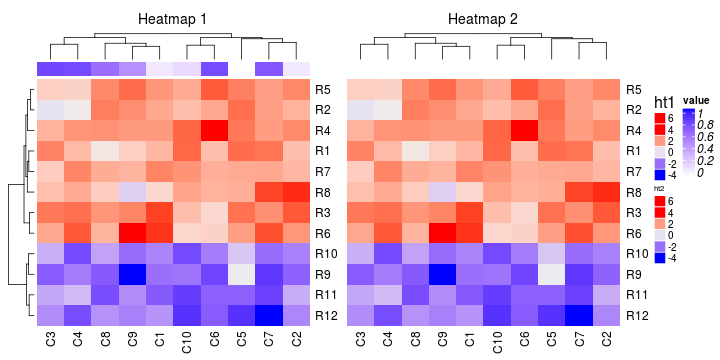``````ht_global_opt(RESET = TRUE)
``````

ComplexHeatmap only generates legends for heatmaps and simple annotations. Self-defined legends can be passed by `heatmap_legend_list` and `annotation_legend_list` as a list of `grob` objects.

grid package provides `legendGrob()` to construct a legend grob with certain style but styles are still limited. For advanced users, they can construct a legend grob totally from ground by `frameGrob()` and `placeGrob()`.

``````ha = HeatmapAnnotation(points = anno_points(rnorm(10)))
ht2 = Heatmap(mat, name = "ht2", column_title = "Heatmap 2", top_annotation = ha, show_heatmap_legend = FALSE)
lgd = legendGrob(c("dots"), pch = 16)
draw(ht1 + ht2, annotation_legend_list = list(lgd))
``````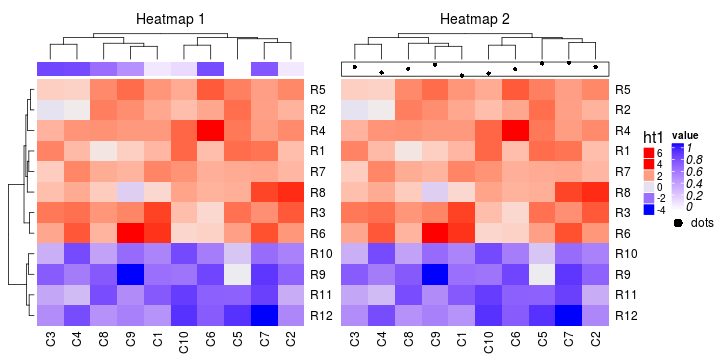From version 1.9.7, ComplexHeatmap package provides a `Legend()` function which can produce legends in `grob` formats (actually all legends in the package are implemented by `Legend()` function). In following example, we have several column annotations which contains points and we also want to show legends for these non-heatmap graphics.

``````ha = HeatmapAnnotation(points = anno_points(rnorm(10), gp = gpar(col = rep(2:3, each = 5))))
ht = Heatmap(mat, name = "ht2", column_title = "Heatmap 2", top_annotation = ha)
lgd = Legend(at = c("class1", "class2"), title = "points", type = "points", legend_gp = gpar(col = 2:3))
draw(ht, annotation_legend_list = list(lgd))
``````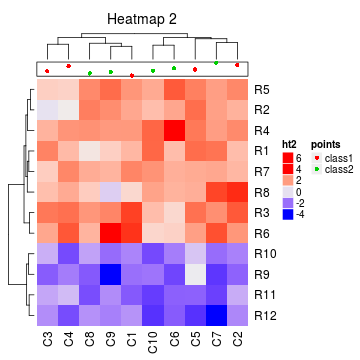Also check this blog link for more demonstrations.

## Session info

``````sessionInfo()
``````
``````## R version 3.4.0 (2017-04-21)
## Platform: x86_64-pc-linux-gnu (64-bit)
## Running under: Ubuntu 16.04.2 LTS
##
## Matrix products: default
## BLAS: /home/biocbuild/bbs-3.5-bioc/R/lib/libRblas.so
## LAPACK: /home/biocbuild/bbs-3.5-bioc/R/lib/libRlapack.so
##
## locale:
##   LC_CTYPE=en_US.UTF-8       LC_NUMERIC=C               LC_TIME=en_US.UTF-8
##   LC_COLLATE=C               LC_MONETARY=en_US.UTF-8    LC_MESSAGES=en_US.UTF-8
##  LC_TELEPHONE=C             LC_MEASUREMENT=en_US.UTF-8 LC_IDENTIFICATION=C
##
## attached base packages:
##   stats4    parallel  grid      stats     graphics  grDevices utils     datasets  methods
##  base
##
## other attached packages:
##   dendextend_1.5.2      dendsort_0.3.3        cluster_2.0.6         HilbertCurve_1.6.0
##   GenomicRanges_1.28.0  GenomeInfoDb_1.12.0   IRanges_2.10.0        S4Vectors_0.14.0
##   BiocGenerics_0.22.0   circlize_0.3.10       ComplexHeatmap_1.14.0 knitr_1.15.1
##  markdown_0.8
##
## loaded via a namespace (and not attached):
##   fastcluster_1.1.22      shape_1.4.2             modeltools_0.2-21       GetoptLong_0.1.6
##   kernlab_0.9-25          lattice_0.20-35         colorspace_1.3-2        viridisLite_0.2.0
##   prabclus_2.2-6          RColorBrewer_1.1-2      fpc_2.1-10              GenomeInfoDbData_0.99.0
##  plyr_1.8.4              robustbase_0.92-7       stringr_1.2.0           zlibbioc_1.22.0
##  munsell_0.4.3           gtable_0.2.0            GlobalOptions_0.0.11    mvtnorm_1.0-6
##  evaluate_0.10           flexmix_2.3-13          class_7.3-14            highr_0.6
##  DEoptimR_1.0-8          trimcluster_0.1-2       Rcpp_0.12.10            scales_0.4.1
##  diptest_0.75-7          XVector_0.16.0          mime_0.5                gridExtra_2.2.1
##  rjson_0.2.15            ggplot2_2.2.1           png_0.1-7               stringi_1.1.5
##  tools_3.4.0             bitops_1.0-6            HilbertVis_1.34.0       magrittr_1.5
##  lazyeval_0.2.0          RCurl_1.95-4.8          tibble_1.3.0            whisker_0.3-2
##  MASS_7.3-47             viridis_0.4.0           mclust_5.2.3            nnet_7.3-12
##  compiler_3.4.0
``````